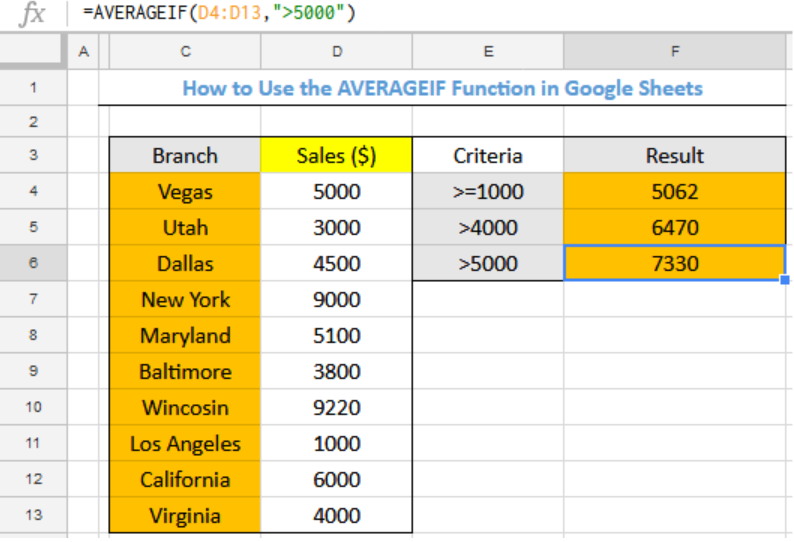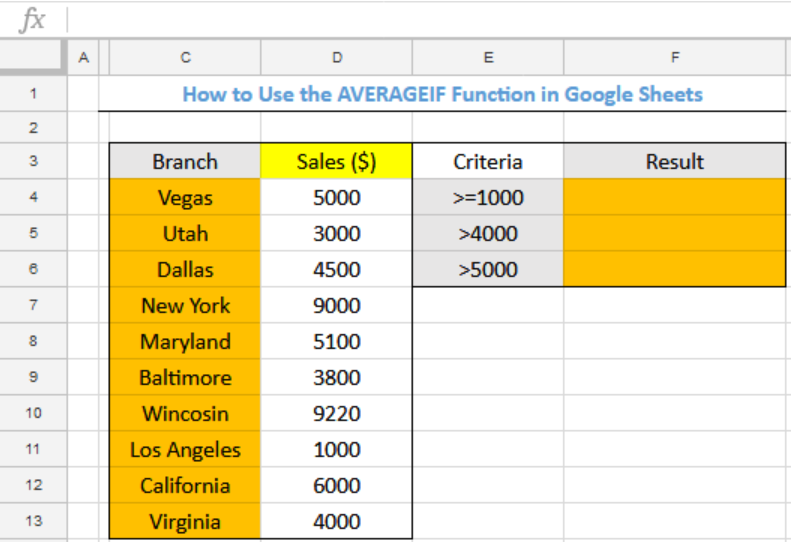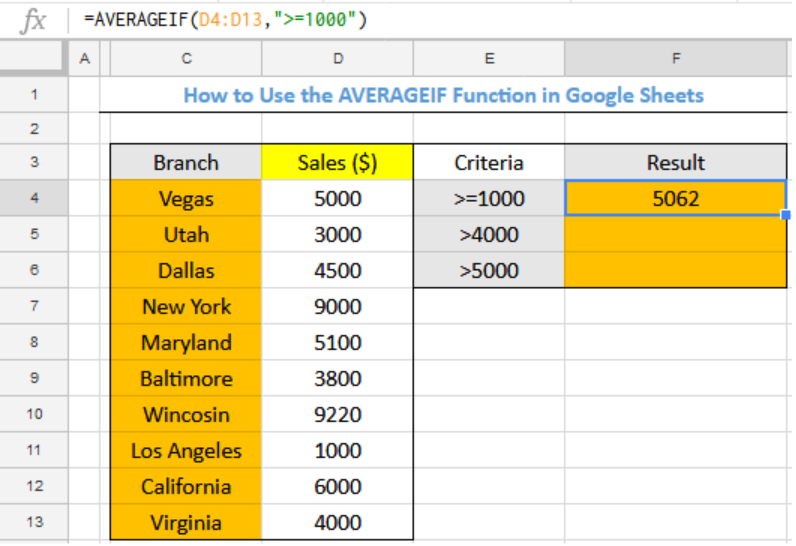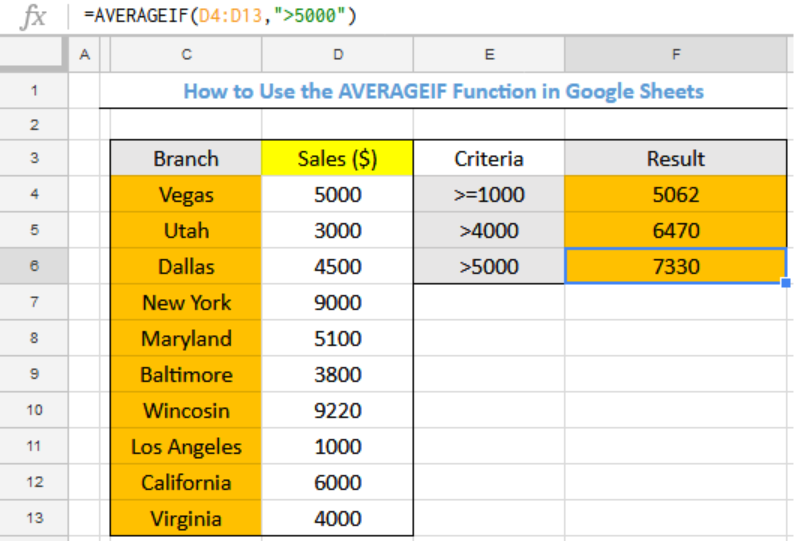Get instant live expert help with Excel or Google Sheets“My Excelchat expert helped me in less than 20 minutes, saving me what would have been 5 hours of work!”

#### Post your problem and you’ll get expert help in seconds.

Your message must be at least 40 characters
Our professional experts are available now. Your privacy is guaranteed.

# Learn How to Use the AVERAGEIF Function in Google Sheets

We can use the AVERAGEIF function to calculate the average of numbers in a range that meet the specified criteria in a Google Sheet. Logical operators like >, <, <>, = and wildcards (*,?) for partial matching are supported by the criteria of AVERAGEIF. The steps below will walk through the process.Figure 1- How to Use the AVERAGEIF Function in Google Sheets

## Syntax

`=AVERAGEIF(range,criteria,[average_range])`

• Range: This could be one or more cells, a named range, arrays, or references.
• Criteria: This could be a number, an expression, cell reference, or text.

• Average_range [optional]: These are the cells to get the average. If we omit it, the range is used.

## Formula

Greater than or equal to 1000: `=AVERAGEIF(D4:D13,">=1000")`

Greater than 4000: `=AVERAGEIF(D4:D13,">4000")`

Greater than 5000: `=AVERAGEIF(D4:D13,">5000")`

## Setting up the Data

• The Branches of a store will be entered into Column C
• Column D will contain the Sales for each of the stores
• The result will be returned in Column F with the specified criteria in Column EFigure 2 – Setting up the Data

## AVERAGEIF IF Sales is >=1000

• We will click on Cell F4
• We will insert the formula below into Cell F4
`=AVERAGEIF(D4:D13,">=1000")`
• We will press the enter keyFigure 3- AVERAGEIF if Sales is >=1000

In a similar fashion, we will enter the formula below into Cell F5 and Cell F6 to get the result

• Cell F5: `=AVERAGEIF(D4:D13,">4000")`
• Cell F6: `=AVERAGEIF(D4:D13,">5000")`Figure 4- Result of applying the AVERAGEIF function

## Note

• Any cell in a range that contains arguments like TRUE or FALSE are ignored
• Any empty cell within the range is ignored in range and average_range with the AVERAGEIF Function
• The error value #DIV/0! will be returned if there aren’t cells in the range that meet the criteria

## Instant Connection to an Expert through our Excelchat Service

Most of the time, the problem you will need to solve will be more complex than a simple application of a formula or function. If you want to save hours of research and frustration, try our live Excelchat service! Our Excel Experts are available 24/7 to answer any Excel question you may have. We guarantee a connection within 30 seconds and a customized solution within 20 minutes.

Are you still looking for help with the Average function? View our comprehensive round-up of Average function tutorials here.

Solution examplesCould you help me by explaining the IF function?
Solved by G. L. in 24 minsI have a question about Conditional Formatting. =IF('Sheet1'!A1="X",IF('Sheet1'!B1="Y", TRUE, FALSE),FALSE) the set the cell background to red. I would like to make it so that if Sheet1'!B1="Z" I set the back to blue. How can I achieve this.
Solved by B. F. in 39 minsI need to find out if the 2018 yearly sales goals were met if the yearly sales were \$25,000 or more using an IF logical function and to set the formula to return a value of YES if met, and NO if not
Solved by A. A. in 18 minsI filtered data in column A (it is labeled ID #) of a data set. On my next worksheet, column A is also the ID #, but it is a different data set. I want to filter the ID #'s the same for the two sheets, but how do I do that being that I am working with two different sets of data?
Solved by V. H. in 22 minsi want to create a very simple if function in a cell which is, if one cell is less than 1250, then the automatic value in the current cell is 250, dead easy, for someone who knows! Thank you.
Solved by A. H. in 14 mins